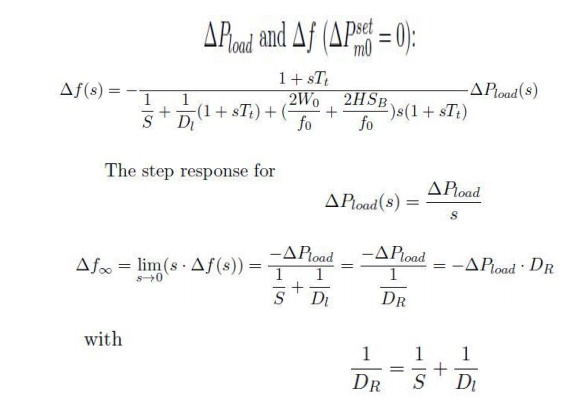Home | | Power System Operation and Control | LFC Control of Single Area and Derive the Dynamic Response

# LFC Control of Single Area and Derive the Dynamic Response

Dynamic Response of the One-Area System

LFC CONTROL OF SINGLE AREA AND DERIVE THE DYNAMIC RESPONSE

## Dynamic Response of the One-Area System

`Now we are going to study the effect of a disturbance in the system derived above. Both loss of generation and loss of load can be simulated by imposing a positive or negative step input on the variable Pload. A change of the set value of the system frequency f0 is not considered as this is not meaningful in real power systems. From the block diagram in Figure.In order to calculate an equivalent time constant Teq, Tt is put to 0. This can be done since for realistic systems the turbine controller time constant Tt is much smaller than the time constantStudy Material, Lecturing Notes, Assignment, Reference, Wiki description explanation, brief detail
Power System Operation and Control : Real Power Frequency Control : LFC Control of Single Area and Derive the Dynamic Response |